### Representations for Lines and Curves

[Hill: p. 555-560]
A preliminary step to drawing lines and curves is choosing a suitable representation for them. There are three possible choices which are potentially useful.
##### Explicit: y = f(x)
 line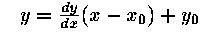circle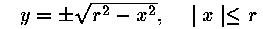##### Parametric:   x = f(t),   y = f(t)
 line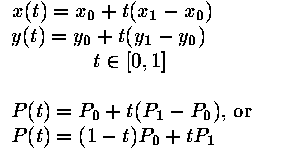circle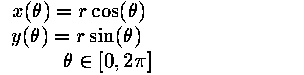##### Implicit:  f(x,y) = 0
 line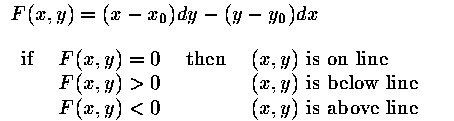circle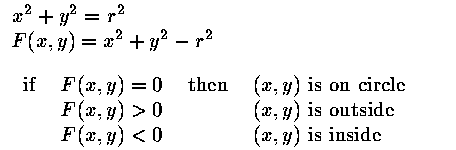### Optimal Line Drawing

Drawing lines on a raster grid implicitly involves approximation. The general process is called rasterization or scan-conversion. What is the best way to draw a line from the pixel (x1,y1) to (x2,y2)? Such a line should ideally have the following properties.
```
```
 straight pass through endpoints smooth independent of endpoint order uniform brightness brightness independent of slope efficient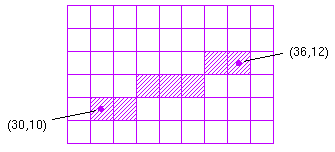##### A Straightforward Implementation
```DrawLine(x1,y1,x2,y2)
int x1,y1,x2,y2;
{
float y;
int x;

for (x=x1; x<=x2; x++) {
y = y1 +  (x-x1)*(y2-y1)/(x2-x1)
SetPixel(x, Round(y) );
}
}```
##### A Better Implementation
```DrawLine(x1,y1,x2,y2)
int x1,y1,x2,y2;
{
float m,y;
int dx,dy,x;

dx = x2 - x1;
dy = y2 - y1;
m = dy/dx;
y = y1 + 0.5;
for (x=x1; x<=x2; x++) {
SetPixel(x, Floor(y) );
y = y + m;
}
}```
```

```
##### The Midpoint Algorithm
The midpoint algorithm is even better than the above algorithm in that it uses only integer calculations. It treats line drawing as a sequence of decisions. For each pixel that is drawn, the next pixel will be either E or NE, as shown below.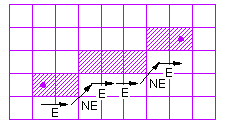The midpoint algorithm makes use of the the implicit definition of the line, F(x,y) = 0. The E/NE decisions are made as follows.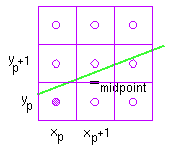define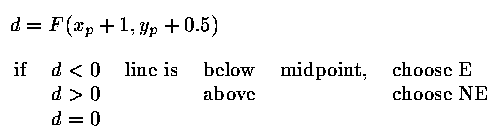if E is chosen,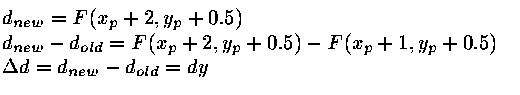if NE is chosen,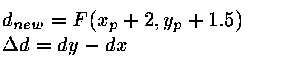The process repeats, stepping along x, making decisions whether to set E or NE pixel.
```

```

#### Initialization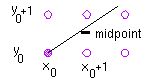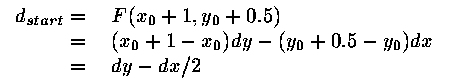Now we would like an integer-only algorithm.
Define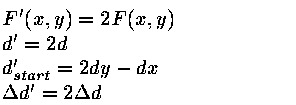##### Midpoint Algorithm
The following algorithm produces the desired results for lines having x1 less than x2 and a slope less or equal to 1.
```drawline(x1, y1, x2, y2, colour)
int x1, y1, x2, y2, colour;
{
int dx, dy, d, incE, incNE, x, y;

dx = x2 - x1;
dy = y2 - y1;
d = 2*dy - dx;
incE = 2*dy;
incNE = 2*(dy - dx);
y = y1;
for (x=x1; x<=x2; x++) {
setpixel(x, y, colour);
if (d>0) {
d = d + incNE;
y = y + 1;
} else {
d = d + incE;
}
}
}```